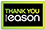# Mathematical Logic

Hardback
Categories: Mathematical Logic
This book gathers together a colorful set of problems on classical Mathematical Logic, selected from over 30 years of teaching. The initial chapters start with problems from supporting fields, like set theory (ultrafilter constructions), full-information game theory (strategies), automata, and recursion theory (decidability, Kleene's theorems). The work then advances toward propositional logic (compactness and completeness, resolution method), followed by first-order logic, including quantifier elimination and the Ehrenfeucht- Fraïssé game; ultraproducts; and examples for axiomatizability and non-axiomatizability. The Arithmetic part covers Robinson's theory, Peano's axiom system, and Gödel's incompleteness theorems. Finally, the book touches universal graphs, tournaments, and the zero-one law in Mathematical Logic. Instructors teaching Mathematical Logic, as well as students who want to understand its concepts and methods, can greatly benefit from this work. The style and topics have been specially chosen so that readers interested in the mathematical content and methodology could follow the problems and prove the main theorems themselves, including Gödel's famous completeness and incompleteness theorems. Examples of applications on axiomatizability and decidability of numerous mathematical theories enrich this volume.
€76.99230 Reward Points
In stock online
Delivery in 2-3 working days

Any purchases for more than €10 are eligible for free delivery anywhere in the UK or Ireland!

This book gathers together a colorful set of problems on classical Mathematical Logic, selected from over 30 years of teaching. The initial chapters start with problems from supporting fields, like set theory (ultrafilter constructions), full-information game theory (strategies), automata, and recursion theory (decidability, Kleene's theorems). The work then advances toward propositional logic (compactness and completeness, resolution method), followed by first-order logic, including quantifier elimination and the Ehrenfeucht- Fraïssé game; ultraproducts; and examples for axiomatizability and non-axiomatizability. The Arithmetic part covers Robinson's theory, Peano's axiom system, and Gödel's incompleteness theorems. Finally, the book touches universal graphs, tournaments, and the zero-one law in Mathematical Logic. Instructors teaching Mathematical Logic, as well as students who want to understand its concepts and methods, can greatly benefit from this work. The style and topics have been specially chosen so that readers interested in the mathematical content and methodology could follow the problems and prove the main theorems themselves, including Gödel's famous completeness and incompleteness theorems. Examples of applications on axiomatizability and decidability of numerous mathematical theories enrich this volume.
Quantity:
In stock online
Delivery in 2-3 working days230 Reward Points

Any purchases for more than €10 are eligible for free delivery anywhere in the UK or Ireland!

€76.99
In stock online
Delivery in 2-3 working days